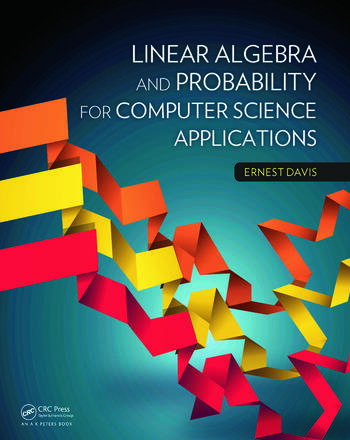# Linear Algebra and Probability for Computer Science Applications

## 1st Edition

Ernest Davis

A K Peters/CRC Press
Published May 2, 2012
Textbook - 431 Pages
ISBN 9781466501553 - CAT# K14397

For Instructors Request Inspection Copy

For Instructors and Students Companion Website >>

was \$72.95

USD\$58.36

SAVE ~\$14.59

FREE Standard Shipping!

## Preview

### Summary

Based on the author’s course at NYU, Linear Algebra and Probability for Computer Science Applications gives an introduction to two mathematical fields that are fundamental in many areas of computer science. The course and the text are addressed to students with a very weak mathematical background. Most of the chapters discuss relevant MATLAB® functions and features and give sample assignments in MATLAB; the author’s website provides the MATLAB code from the book.

After an introductory chapter on MATLAB, the text is divided into two sections. The section on linear algebra gives an introduction to the theory of vectors, matrices, and linear transformations over the reals. It includes an extensive discussion on Gaussian elimination, geometric applications, and change of basis. It also introduces the issues of numerical stability and round-off error, the discrete Fourier transform, and singular value decomposition. The section on probability presents an introduction to the basic theory of probability and numerical random variables; later chapters discuss Markov models, Monte Carlo methods, information theory, and basic statistical techniques. The focus throughout is on topics and examples that are particularly relevant to computer science applications; for example, there is an extensive discussion on the use of hidden Markov models for tagging text and a discussion of the Zipf (inverse power law) distribution.

Examples and Programming Assignments
The examples and programming assignments focus on computer science applications. The applications covered are drawn from a range of computer science areas, including computer graphics, computer vision, robotics, natural language processing, web search, machine learning, statistical analysis, game playing, graph theory, scientific computing, decision theory, coding, cryptography, network analysis, data compression, and signal processing.

Homework Problems
Comprehensive problem sections include traditional calculation exercises, thought problems such as proofs, and programming assignments that involve creating MATLAB functions.

#### Instructors

We provide complimentary e-inspection copies of primary textbooks to instructors considering our books for course adoption.